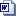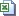Nuffield Mathematics teaching resources are for use in secondary and further education

# Level 3 Calculus

## What's it worth?

Students solve differential equations to find functions to model the value of a car in terms of its age. Then they compare their results with real data.

## Exponential rates of change

This activity introduces the differentiation of exponential functions. Students find gradients by drawing tangents and by using small incremental changes in a spreadsheet.

## Stationary points

This activity shows students how to use differentiation to find stationary points on the curves of polynomial functions. It includes the use of to determine the nature of the stationary point. Students will learn how to use this and other information to sketch the curves, then use graphic calculators to check their answers.

## Maxima and mimina

Students use differentiation to find maximum and minimum points in polynomial functions in order to solve real-life problems.

## Maximising and minimising

Slideshow to aid class discussion about solving maximisation and minimisation problems. The slideshow then encourages students to devise and solve their own problems. There are no student sheets.

## Coastal erosion A Estimating area

Students approximate the area of a piece of land with an irregular coastline, and the area lost to coastal erosion over time.Slides (1.4 MB)Student sheets Word 2007 (232 KB)Student sheets pdf (582 KB)Teacher notes Word (194 KB)Teacher notes pdf (391 KB)

## Coastal erosion B Integrating area

Students use integration to approximate the area of a piece of land with an irregular coastline, and the area lost to coastal erosion over time.Slides (1.4 MB)Spreadsheet (142 KB)Student sheets Word 2007 (247 KB)Student sheets pdf (603 KB)Teacher notes Word (183 KB)Teacher notes pdf (383 KB)

## Drug clearance

This activity explores the rate of drug clearance from the body, both for common analgesic compounds and for caffeine.Slides (1.3 MB)Student sheets Word (175 KB)Student sheets pdf (539 KB)Teacher notes Word 2007 (175 KB)Teacher notes pdf (424 KB)

## Derivative matching

Matching activities to check whether students can identify the derivatives of quadratic and cubic functions and their graphs.

## Mean values

Students learn how to find the mean values of quantities varying with time. This involves finding the area under graphs, initially by using geometrical formulae for areas. It is followed by the use of integration in a variety of real life situations.SlidesStudent sheets WordStudent sheets pdfTeacher notes Word 2007Teacher notes pdf